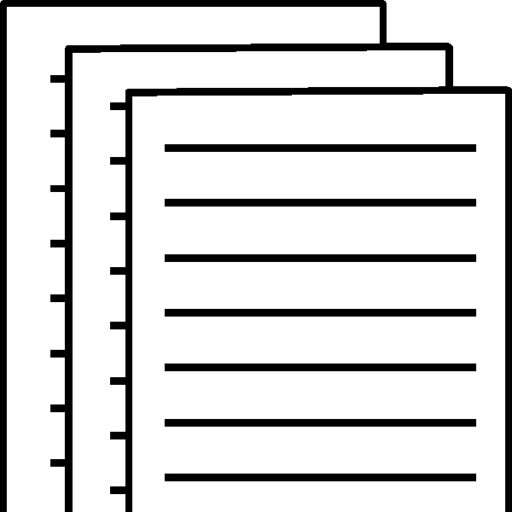## HCS 380 week 3

Question 1

The following are financial statements of Wildhorse Co..

Net sales   \$2,234,400
Cost of goods sold   1,030,500
Interest expense   96,100
Income tax expense   70,590
Net income   \$ 116,810
Assets
Current assets
Cash   \$ 76,000
Debt investments   85,590
Accounts receivable (net)   182,600
Inventory   155,000
Total current assets   499,190
Plant assets (net)   593,400
Total assets   \$ 1,092,590
Liabilities and Stockholders’ Equity
Current liabilities
Accounts payable   \$ 175,900
Income taxes payable   37,090
Total current liabilities   212,990
Bonds payable   212,800
Total liabilities   425,790
Stockholders’ equity
Common stock   368,100
Retained earnings   298,700
Total stockholders’ equity   666,800
Total liabilities and stockholders’ equity   \$1,092,590

Additional information: The net cash provided by operating activities for 2017 was \$206,700. The cash used for capital expenditures was \$110,100. The cash used for dividends was \$32,590. The weighted-average number of shares outstanding during the year was 50,000.

(a) Compute the following values and ratios for 2017. (We provide the results from 2016 for comparative purposes.) (Round Current Ratio and Earnings per share to 2 decimal places, e.g. 15.25 and Debt to assets ratio to 1 decimal place, e.g. 78.9%. Enter negative amounts using either a negative sign preceding the number e.g. -45 or parentheses e.g. (45).)
(i) Working capital. (2016: \$160,500) Current ratio. (2016: 1.65:1) Free cash flow. (2016: \$48,700) Debt to assets ratio. (2016: 31%) Earnings per share. (2016: \$3.15)
(i) (ii) (iii) Working capital \$ Current ratio :1 Free cash flow \$ Debt to assets ratio % Earnings per share \$

Question 2

The following information is available for Marin Inc. for three recent fiscal years.

 Inventory Net sales Cost of goods sold 2017 2016 2015 \$546,328 \$571,700 \$326,238 1,934,372 1,695,980 1,327,594 1,554,675 1,312,366 964,008

Calculate the inventory turnover, days in inventory, and gross profit rate for 2017 and 2016. (Round inventory turnover to 1 decimal place, e.g. 5.2, days in inventory to 0 decimal places, e.g. 125 and gross profit rate to 1 decimal place, e.g. 5.2%.)

Inventory Turnover Days in Inventory 2017 2016 times times days days % %
 HYPERLINK “javascript:void(0)” o “Collapse question part” (a)Your answer is correct.  Calculate the accounts receivable turnover and the average collection period for 2017 for FedEx Corporation. (Round answers to 1 decimal place, e.g. 12.5. Use 365 days for calculation.)Accounts receivable turnover timesThe average collection period for 2017 daysSHOW LIST OF ACCOUNTSSHOW SOLUTIONSHOW ANSWERLINK TO TEXTAttempts: 1 of 3 used
 HYPERLINK “javascript:void(0)” o “Collapse question part” (b)Your answer is correct.  Is accounts receivable a material component of the company’s total current assets?

Question 4

Carla Vista Co. reports the following information (in millions) during a recent year: net sales, \$10,040.0; net earnings, \$320.0; total assets, ending, \$4,550.0; and total assets, beginning, \$5,475.0.

(a) Calculate the (1) return on assets, (2) asset turnover, and (3) profit margin. (Round answers to 1 decimal place, e.g. 6.2% and 6.2.)

1. 2. Return on assets % Asset turnover times Profit margin %

To view and download a complete answer, scroll down to the bottom to pay